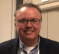## Calculating noise floor of digitization system using FFTStarted by 4 years ago9 replieslatest reply 4 years ago3445 views

Hi all

I am trying to calculate self noise of a digitization system with differential inputs.For this purpose, i select a differential channel, set input range to +/-5 V, short both differential inputs and performed following steps:

1. Collect 'x' no of samples of voltage signal
2. Perform FFT of size 'x' on collected samples
3. Divide each complex output of FFT by 'x'
4. Find the absolute of each complex output of FFT
5. Multiply the output of above step by 1.414 to get Vrms against each frequency bin
6. Take log10 and multiply by 20 of each output in above step
7. Add 10xlog10(x/2) in each output of step above to compensate FFT gain against noise

The result is self noise floor graph of a channel in terms of dBVrms Vs frequency.

Kindly guide me whether this method is accurate enough to estimate self noise of a digitizer?

Thanks

[ - ]Hi Naumankalia,

What is the difference between your scheme and just applying a full-scale sinewave and then taking the windowed fft?  What is your definition of self noise?

Note that in the DSP world, we typically refer to the signal as x(n), where n is the sample number.  Usually the total number of samples is called N.

Regarding computation of the power of a captured signal, see my post at:

https://www.dsprelated.com/showarticle/1004.php

The post includes windowing the signal, and normalizes the window amplitude so that it does not effect the power calculation.

regards,

Neil

[ - ]Thanks for reply. I am sorry i could not clearly explain the purpose of this scheme. My main purpose is to determine practically (not theoretically) Hardware noise of my digitization system (which i call self noise of system) across a frequency band. Reason to do this is to determine the minimum input signal level which will be detectable by digitization system beyond which it will merge in own noise of system.

[ - ]Hello ,

The digitizer probably consists of ADC and DSP, according to your algorithm for noise calculation.

Regarding ADC, the quantization noise within fs/2 is 6*N (ADC number of bits) + 4.77dB relative to the peak voltage, this is HW limitation.

If you do oversampling, you have to take that into account as 10*log(fs/2/BW).

Regarding DSP, it is function of your FFT setting, this computation limitation.

Typically, the computation is negligible relative to HW limitation, if the FFT and Algorithms parameters are set appropriately.

Best regards,

Shahram Shafie

[ - ]Thanks for reply. I am sorry i could not clearly explain the purpose of this scheme. My main purpose is to determine practically (not theoretically) Hardware noise of my digitization system (which i call self noise of system) across a frequency band. Reason to do this is to determine the minimum input signal level which will be detectable by digitization system beyond which it will merge in own noise of system.
[ - ]Hi, Though it's not clear the term 'self noise', i'm assuming you're looking for noise / harmonics content in your signal. if that is the case, you can use the THD + N measurement. if you're not expecting harmonic distortion you can directly go with SNR measurement.

the terms are well defined and illustrated in https://www.analog.com/media/en/training-seminars/...

Do remember the following while you perform FFT:

1. select the right window. especially, if you are using sine tone to measure THD and the freq is not a multiple of sampling rate. using right win will help to keep the bin slim.

2. if you're automatically detecting the freq index based on the FFT bin, make use of three adjacent dominant peaks to decide more 'accurate' freq index. there are several approaches to get this value more accurately.   This would be needed of the sine generated by a source which is Independence on the system you use for measurement.

-Chalil

[ - ]Thanks for reply. I am sorry i could not clearly explain the purpose of this scheme. My main purpose is to determine practically (not theoretically) Hardware noise of my digitization system (which i call self noise of system) across a frequency band. Reason to do this is to determine the minimum input signal level which will be detectable by digitization system beyond which it will merge in own noise of system.
[ - ]you haven't described what your input is apart from saying it is +/- 5V.

If you want to estimate noise created by your ADC system then why not just try zero input(i.e. no signal) first. Next try single tone.

zero input should show what will be added to your signal over any bandwidth you choose.

single tone will tell you about quantisation noise for full scale input swing.

[ - ]